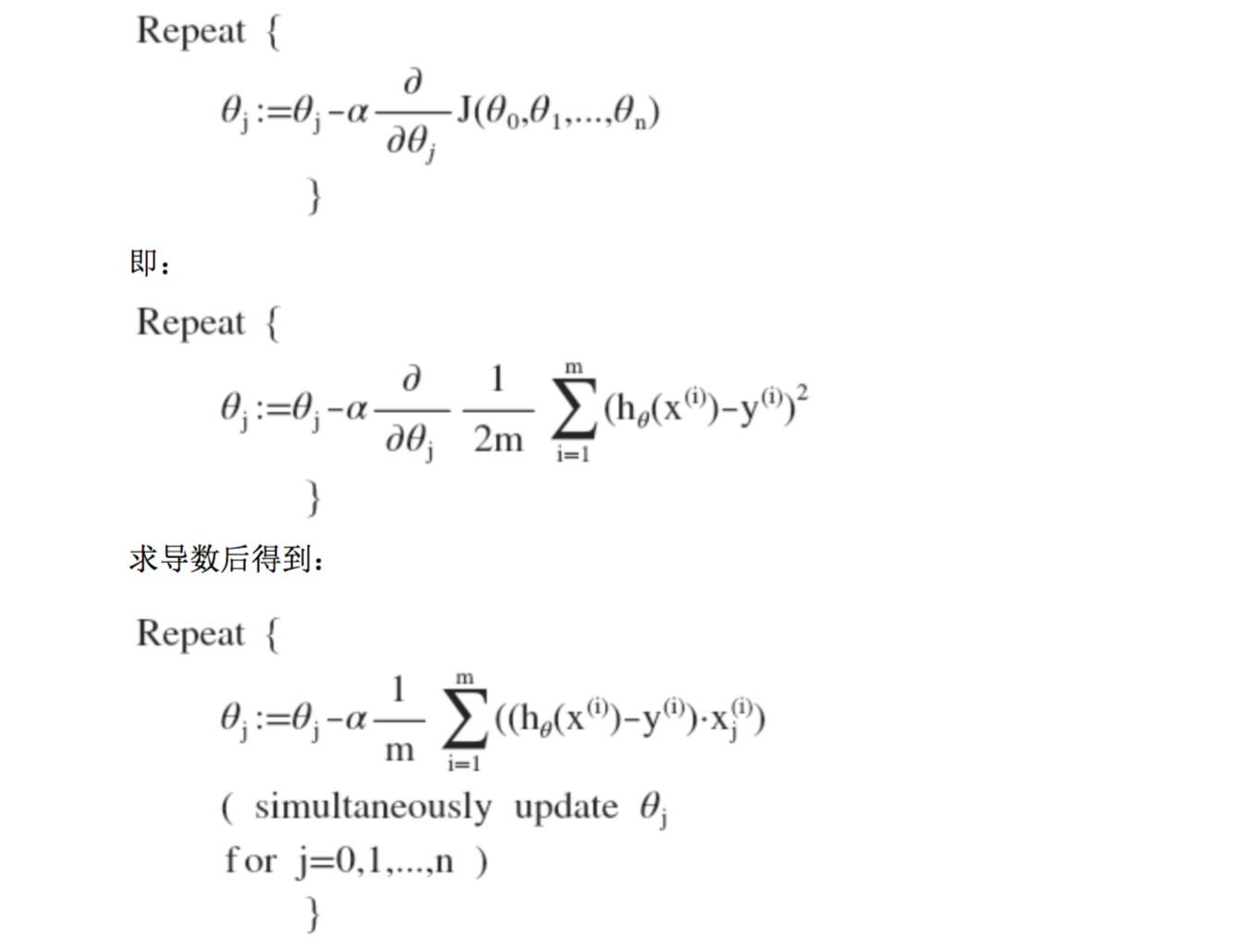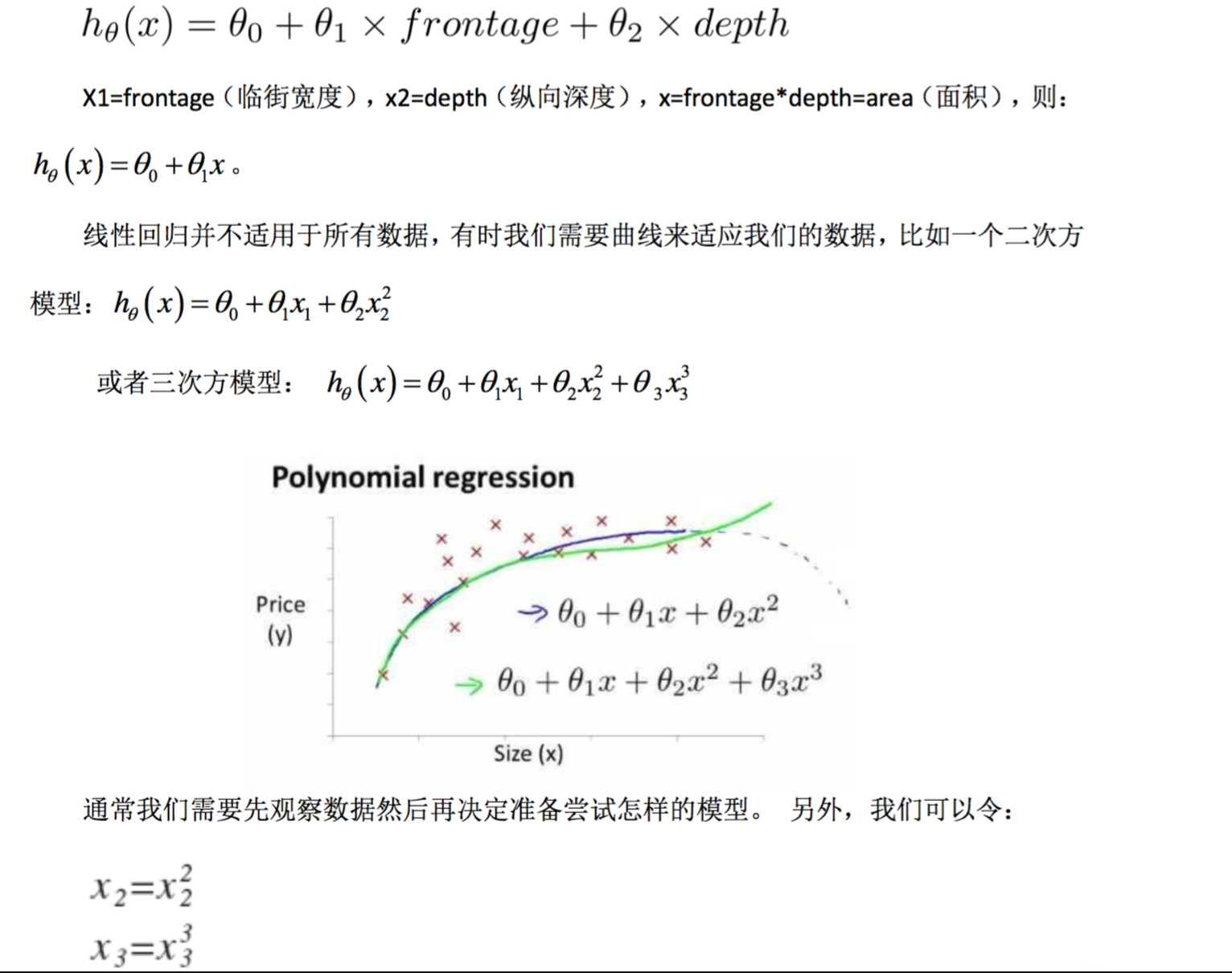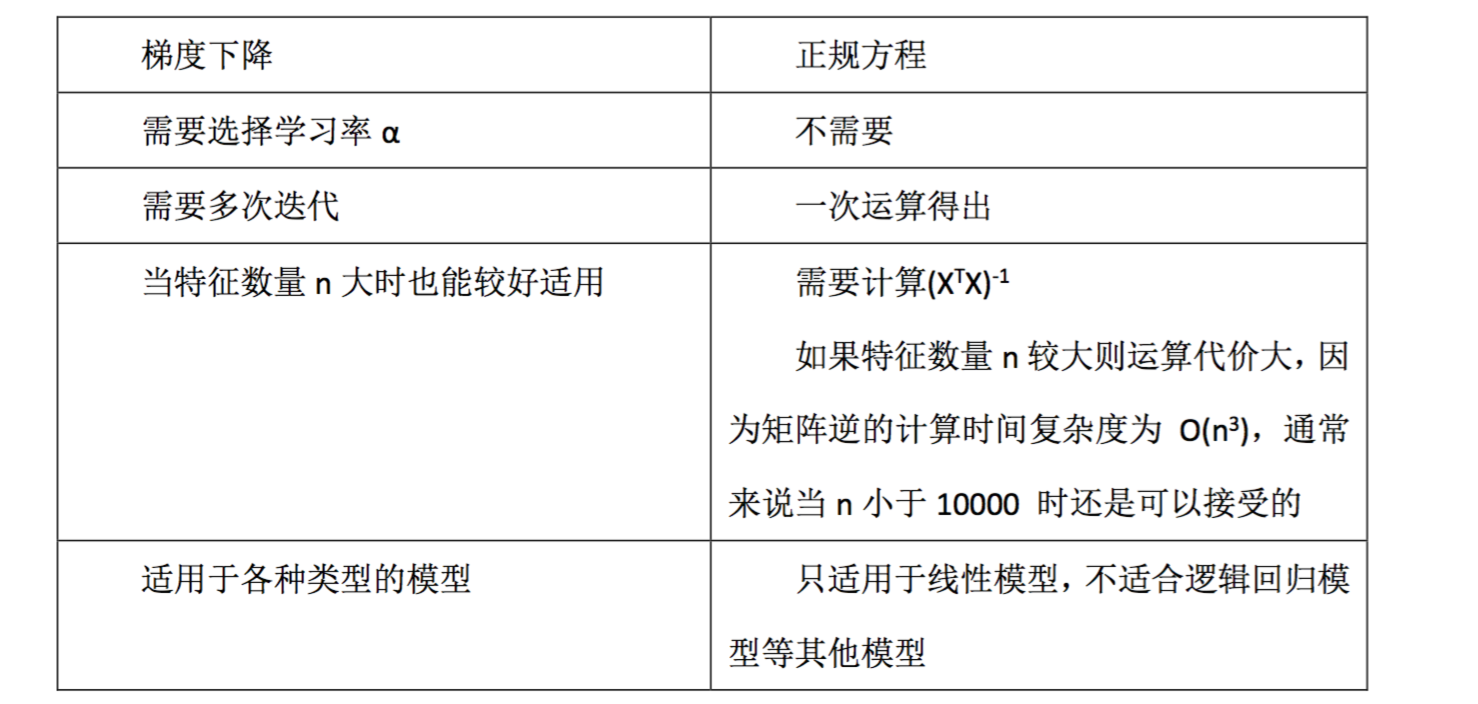# 多变量线性回归

Posted by jjx on November 29, 2016

n 代表特征的数量
$x^{(i)}$代表第 i 个训练实例,是特征矩阵中的第 i 行,是一个向量(vector)。
$x^i_j$ 代表特征矩阵中第 i 行的第 j 个特征,也就是第 i 个训练实例的第 j 个特征。

#### 多变量梯度下降

$j(\theta_0,\theta_1...\theta_n)=\frac{1}{2m}\sum_{i=1}^{m}(h_\theta(x^{(i)})-y^{(i)})^2$$x_n=\frac{x_n-u_n}{s_n}$#### 正规方程

$\Theta=(X^TX)^{-1}X^Ty$